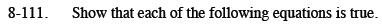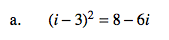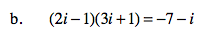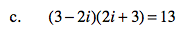Home > CCA2 > Chapter 8 > Lesson 8.2.3 > Problem8-111

8-111.
1. Show that each of the following equations is true. Homework Help ✎

1. (i − 3)2 = 8 − 6i

2. (2i − 1)(3i + 1) = −7 − i

3. (3 − 2i)(2i + 3) = 13Start by multiplying (i − 3)(i − 3). Use a generic rectangle if you need one.

$i^2 - 6i + 9 = 8 - 6i$

$i^2 = -1, \text{ so}\:i^2 - 6i + 9 = -1 - 6i + 9 = 8 - 6i$See part (a).See part (a).